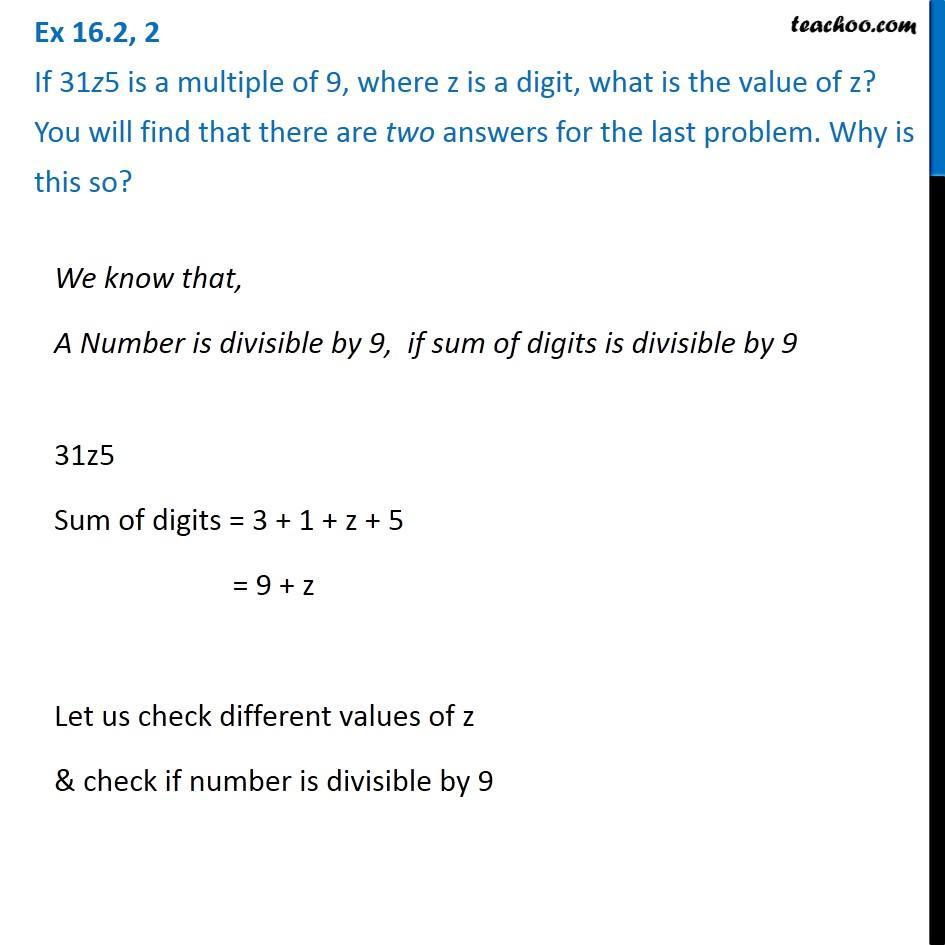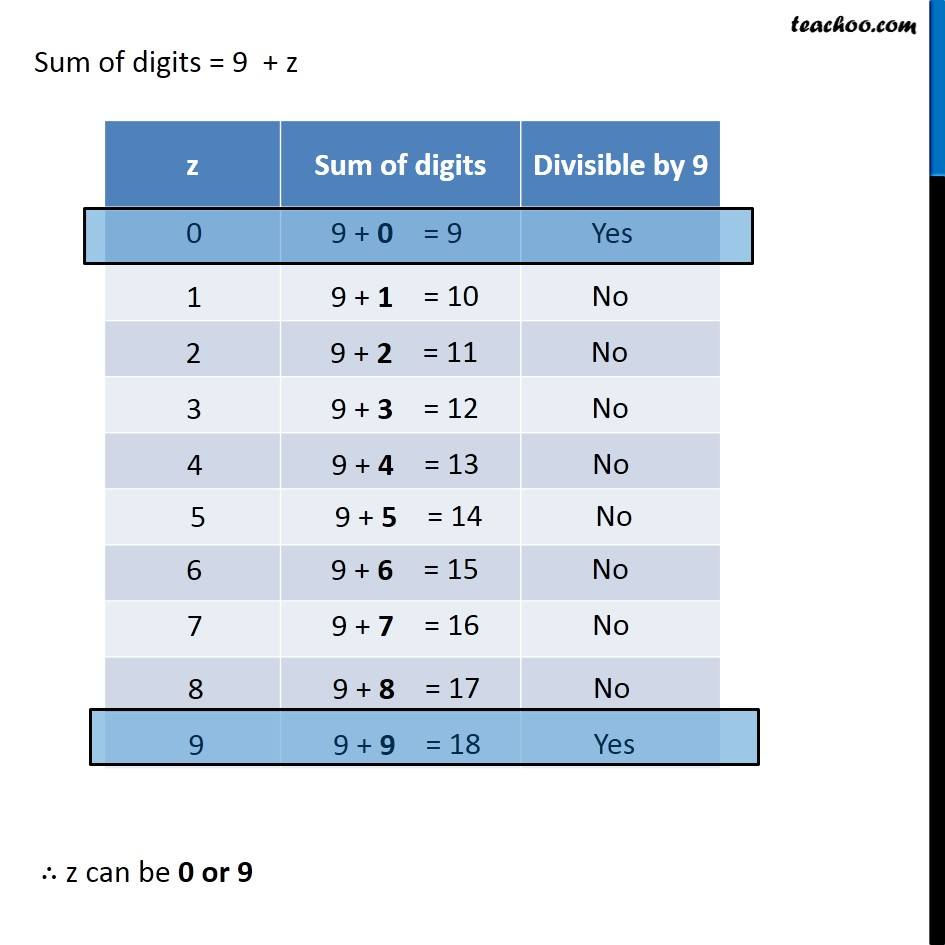Fill in the steps

Playing with Numbers
Serial order wiseLearn in your speed, with individual attention - Teachoo Maths 1-on-1 Class

### Transcript

Question 2 If 31z5 is a multiple of 9, where z is a digit, what is the value of z? You will find that there are two answers for the last problem. Why is this so?We know that, A Number is divisible by 9, if sum of digits is divisible by 9 31z5 Sum of digits = 3 + 1 + z + 5 = 9 + z Let us check different values of z & check if number is divisible by 9 Sum of digits = 9 + z